Courses
Courses for Kids
Free study material
Offline Centres
More

# Identifying Numbers and QuantitiesLast updated date: 30th Nov 2023
Total views: 98.7k
Views today: 1.98k## Introduction to Identifying Numbers and Quantities

Identification of numbers is taught at a very early age to kids. In fact, not only numbers but other things that we see in our daily lives are identified to young kids. In simple terms, number identification is the ability to identify any number that corresponds with quantity. The whole process here is also known as number representation or number recognition.

To identify any object or article a visual representation for kids is quite important. They may learn the numbers but may not quantify them. Further in this article, we will talk about counting and matching numbers, printable numbers in circles, a symbol used for counting, numbers with dots for counting, and a few more.

## Number Identification and Quantities

Number identification is the ability to identify the number that corresponds with a quantity. This is also called number representation or number recognition. For example, it is the ability to identify that the symbol “7” refers to the word “seven” and a quantity of seven objects. In this way, numbers should be identified using their quantities so it becomes easier for kids to learn faster.

In fact, there are many activities to help kids identify numbers. Some of the easiest ways are to put numbers everywhere, use a calculator, use magnetic numbers, use an abacus, and many more. In fact, an abacus is the best way to identify numbers with quantity. These are some of the best activities for kids to learn numbers at an early age.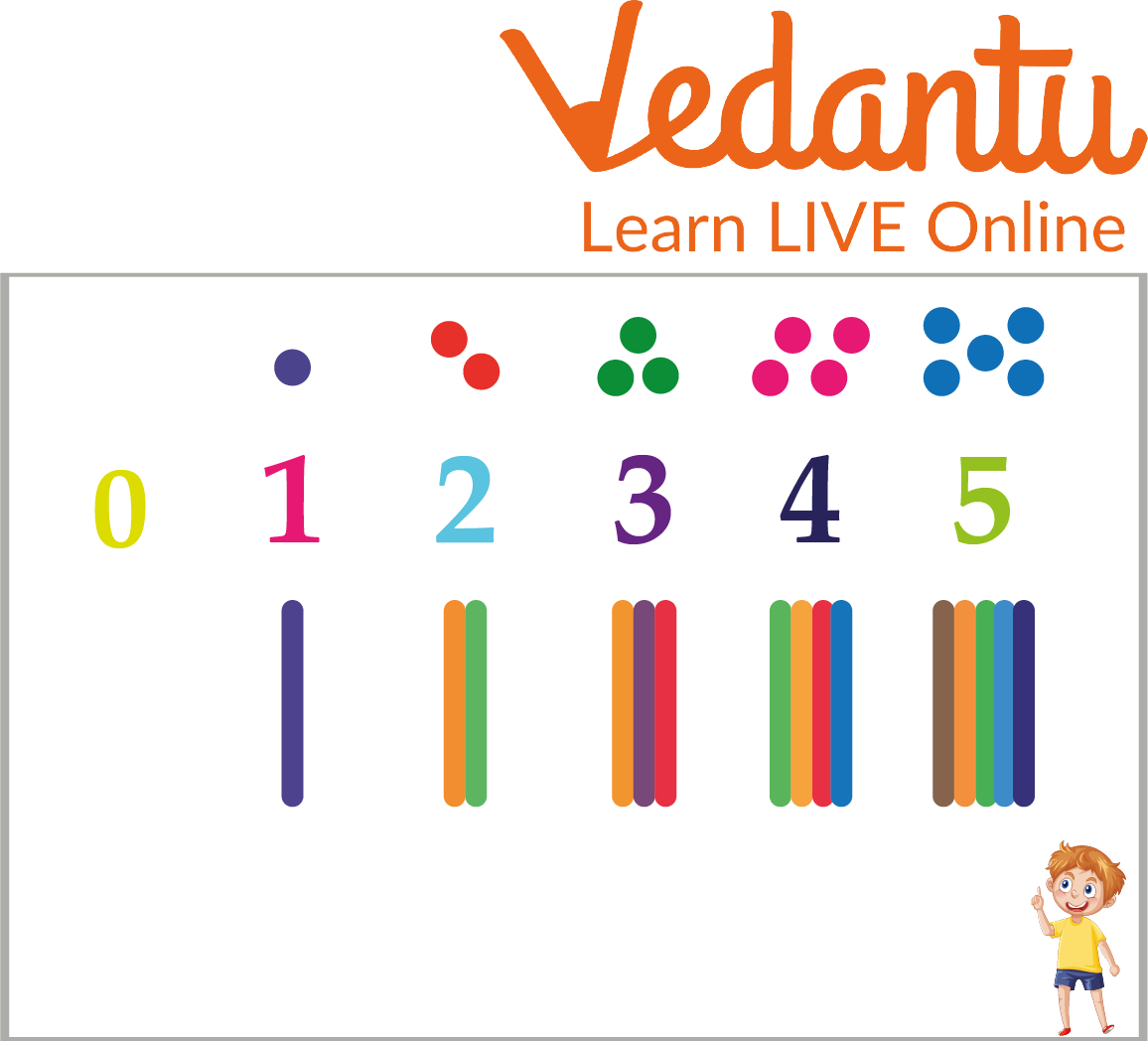Number identification and quantities

## Counting and Matching Numbers

Counting is memorizing numbers in correct order (1, 2, 3, 4, 5…). One-to-one correspondence is the skill of pairing or matching objects so that one object equals one number. That is, when we say, “one,” we count one object and when we say, “two,” we count one more object.

Below is an image where one can see that on one side there are images and on the other side numbers. Interestingly, in the first column there are different fruits but in different quantities. On the other hand, the second column is the numbers column. The kids will be required to match the numbers with the number of fruits given in the first column.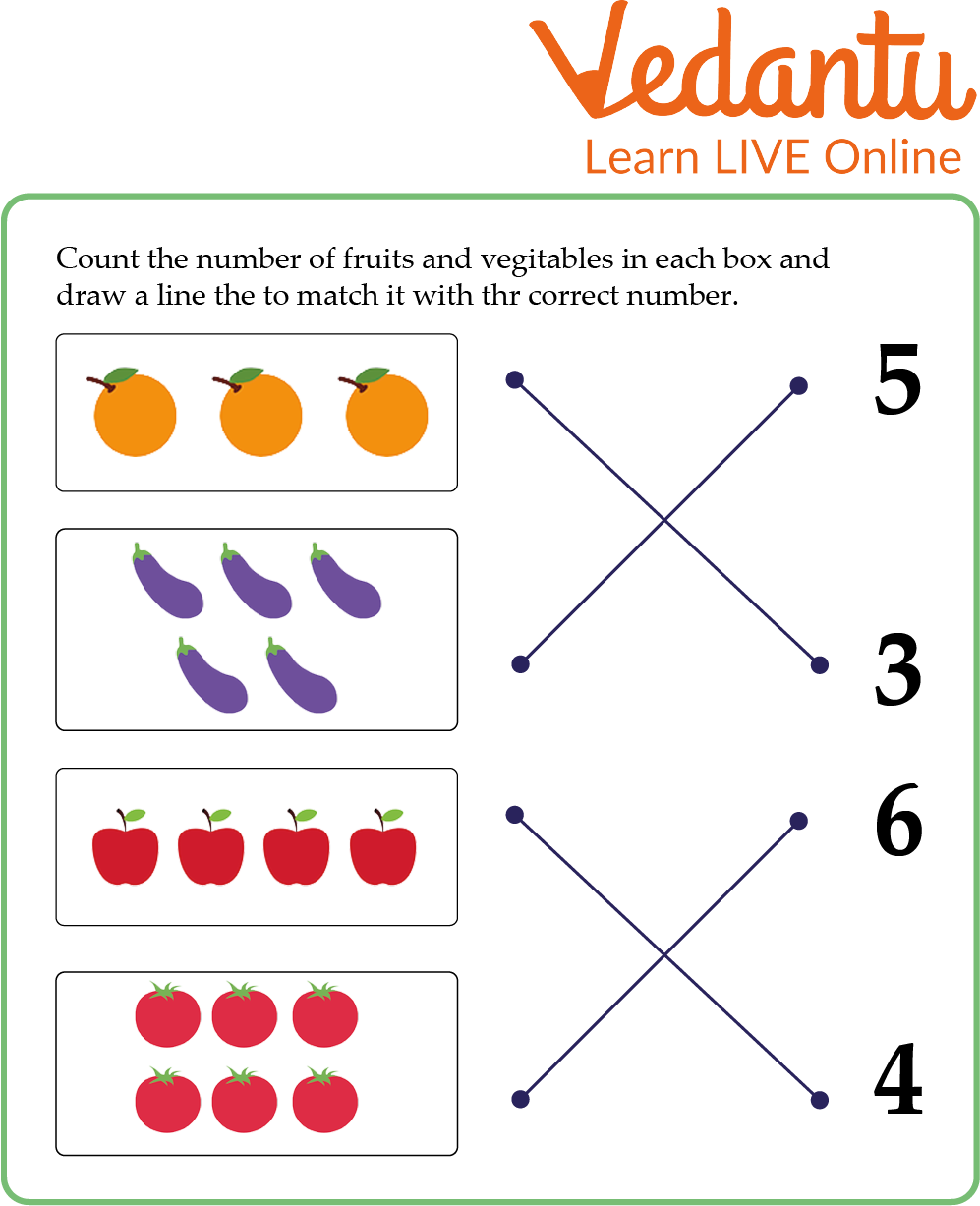Counting and matching numbers

The answers are already given here so that it becomes easier for the kids to understand.

In the first column, we can see there are three fruits that will match with number 3 in the other column. In the second option of the first column, we can see five fruits which will match with number 5 on the right column. The process will follow in a similar manner for other options as well.

## Symbols Used for Counting

Before counting any numbers there are a few things that one should keep in mind. In fact, these things are quite important to know before using numbers. Numbers are divided into variables and constants. To solve any type of numerical problem one must know different numbers. The letter (N) is the symbol used for counting.

1. ### Natural Numbers

Natural numbers are counting numbers that begin with 1 and continue to infinity. It is denoted as (N). Example: 1, 2, 3, 4, 5……

1. ### Whole Numbers

The letter (W) represents whole numbers. It is the counting of numbers starting from 0 to infinity. Example: 0, 1, 2, 3, ……

1. ### Integers

Integers are represented with the English alphabet (Z). An integer can be zero, any positive number to infinity, or a negative number to negative infinity. A few examples of integers are: -5, 0, 1, 5, 8, 97, and 3,043.

These are some of the basics that any kid must know before learning about numbers. In fact, these are quite important to solve any problem in the mathematical world.

## Numbers With Dots for Counting

This is a great method for kids to learn new numbers during preschool. In fact, it is one of the most amazing ways to learn numbers and how to write them. There are various printables available for kids in the market. You get printable numbers in a circle book, numbers with dots for counting, numbers for preschool activities, and many more.

Normally, from number 1-10 numbers are hugely printed on this book. Inside these numbers, there are dots that need to be moved from one end to another. This way the kids not only play but learn rapidly how to write and recognize each number.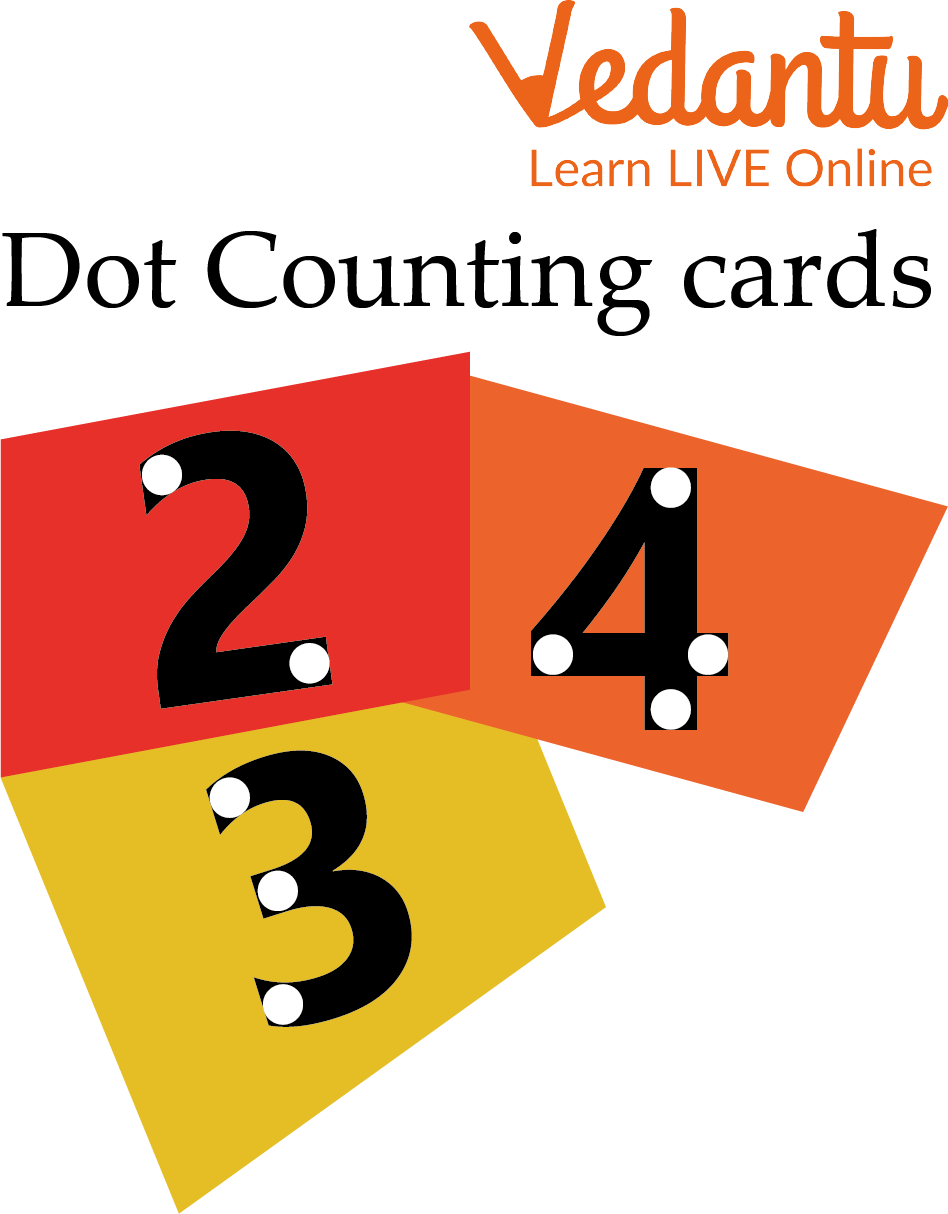Numbers with dots for counting

## Solved Examples

Q 1. Identify the numbers shown in the image:

 Figure Number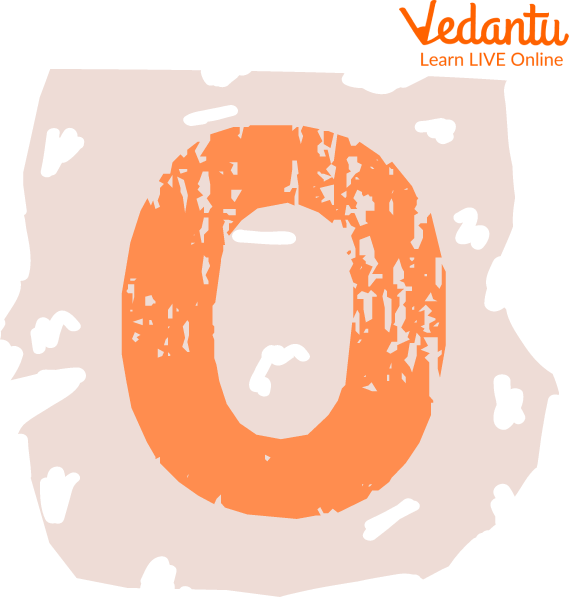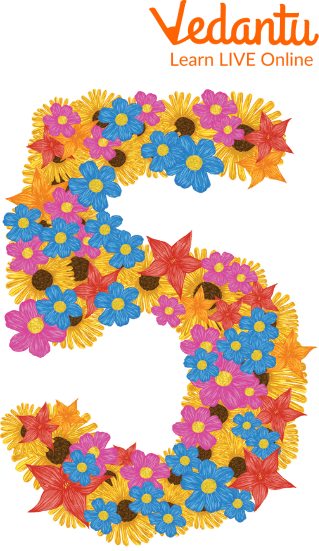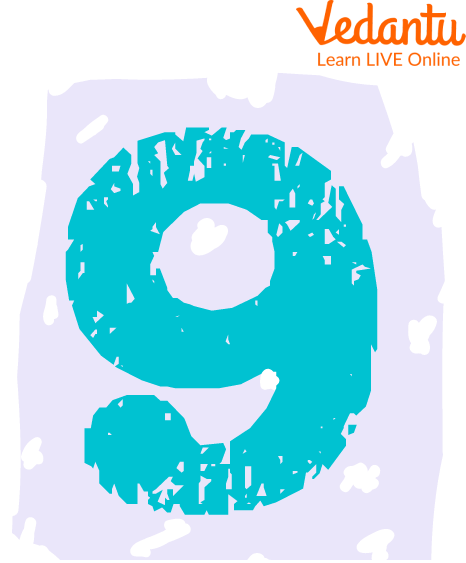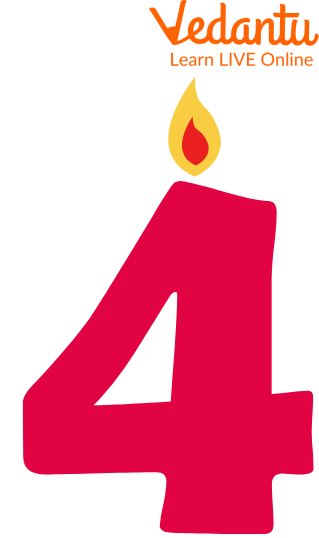Ans: Following are the identified numbers:

 Figure NumberZero (0)Five (5)Six (6)Nine (9)Four (4)

Q 2. There is the set of a number named as N. N = {0, 2, 4, 3, 5, 7, 8, 10}.

Identify the following in it:

(a) Natural number

Ans: Natural number: As we know that, natural numbers are those numbers that start with 1 to infinity. Therefore, 2, 4, 3, 5, 7, 8, 10 are the natural numbers.

(b) Whole number

Ans: Whole number: As we know that, whole numbers are those numbers that start with 0 to infinity. Therefore, 0, 2, 4, 3, 5, 7, 8, 10 are the natural numbers.

## Practice Questions

Q 1. Identify and match the correct numbers to their quantity: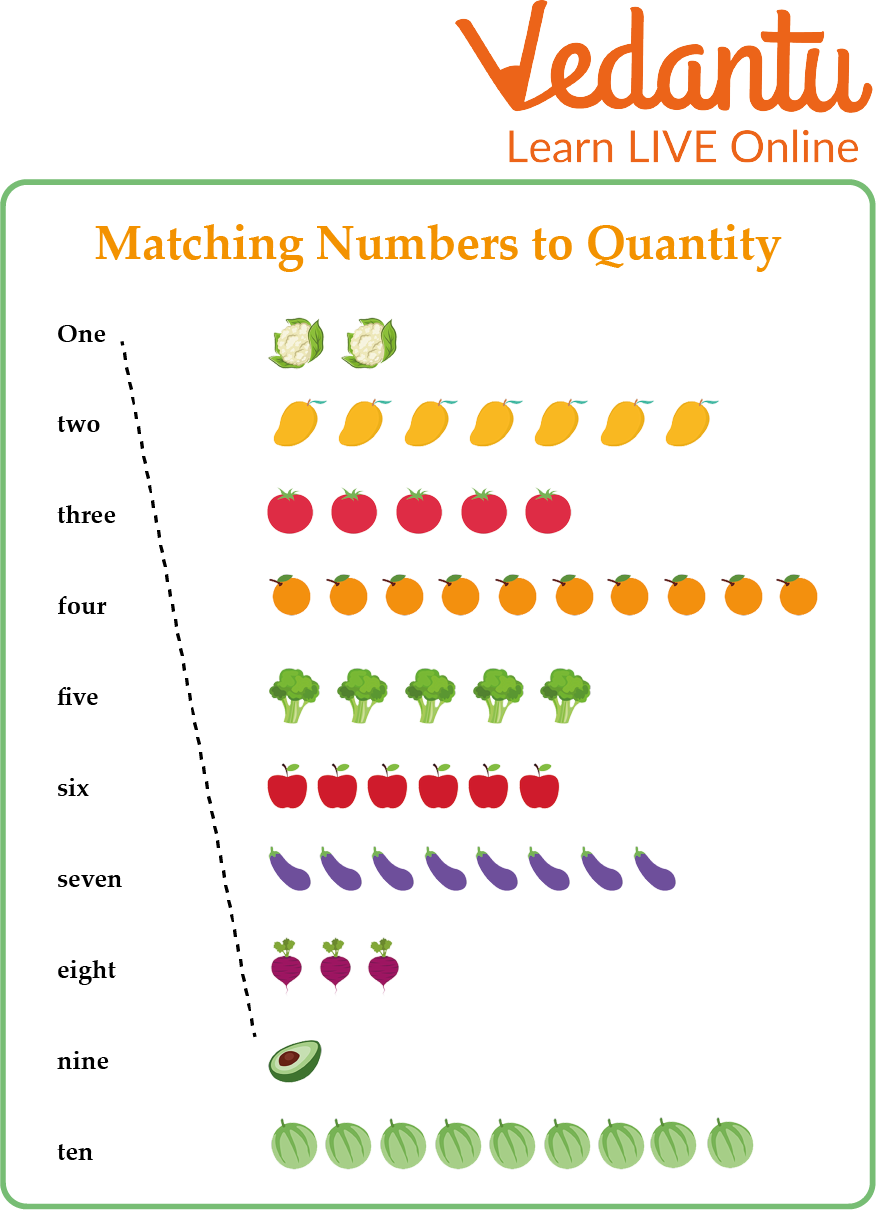Practice Question

Ans: The first is already solved. The answers for rest are given below:

Two- first on the right-hand column

Three- Eighth one on the right-hand column

Four- fifth one on the right-hand column

Five- third on the right-hand column

six - sixth on the right-hand column

Seven- second on the right-hand column

Eight- seventh on the right-hand column

Nine- tenth on the right-hand column

Ten- fourth on the right-hand column

Q 2. Write all the natural numbers between 0 to 25.

Ans: The natural numbers from 0 to 25 are 1, 2, 3, 4, 5, 6, 7, 8, 9, 10, 11, 12, 13, 14, 15, 16, 17, 18, 19, 20, 21, 22, 23, 24.

## Summary

Learning something every day should be a habit for everyone since their childhood. In the same way, learning numbers for kids in such methods would surely be fun. In this article, we have learned about identifying numbers and the quantities. The capacity to recognise the number that corresponds to a quantity is known as number identification. We have added some solved examples, which will help to identify different numbers. To get more clarity, we have also added solved questions. Using these methods and activities the kids will never ever forget to recognize numbers.

## FAQs on Identifying Numbers and Quantities

1. What do children learn from matching numbers through these books?

Matching numbers from a very young age develop representation and problem-solving skills. It is also good for developing fine and motor skills in the kids.

2. What are some preschool activities for kids?

When it comes to numbers preschool activities, there is a long list of them. Some of the famous activities are the following:-

• Roll the dice

• Scavenger hunt

• Graph legos

• Activity books

• Counting objects from nature

3. What is the meaning of the number concept of preschool?

This means the whole concept of understanding numbers in young kids. Doing this helps the kids to understand the connection between quantities, numbers, countings, and many more.